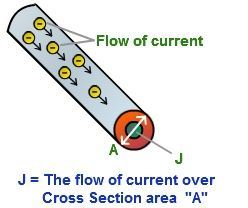# Current Density Formula

The measure of the amount of electric charge per unit time that flows through the unit area of the cross-section. The Current density is a vector quantity. Therefore, it has both magnitude and direction. The S.I unit of Current density is A/mand is denoted by the symbol J.## Formula of Current Density

Current Density Formula is expressed as,

J = I / A

Where,

• I denote the current flowing through the conductor in Amperes
• A denotes the cross-section area in m2
• Current density is expressed in A/m2

### Solved Examples

Example 1

A 5 mm2 copper wire has a current of 5 mA of current flowing through it. Determine the current density.

Solution

Given:

Total Current I is 5 mA

Total Area A is 5 mm2

The Current density  J = I / A

= 5×10−3 / 5×10−3

= 1 A/m2

Example 2

Determine the current density of if 50 Amperes of current flows through the battery in an area of 10 m2?

Solution

Given:

Current I is 50 A,

Area A is 10 m2

The current density is given by J = 50 / 10

J= 5 A/m2.

For more such valuable equations and formulas stay tuned with BYJU’S!!